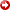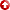[First Hit]4-DNonlinear-Dynamical Fluvial Modeling Using Deterministic Equations and Fuzzy Logic

EDINGTON, DWAINE H. and TIMOTHY A. CROSS

A four-dimensionalnonlinear, dynamical forward model of fluvial stratigraphy, FLUVSIM, has been developed. This program incorporates the following features: 1) The program is deterministic in that it employs empirically developed geomorphic and stratigraphic process-response relationships. It does not use randomly generated processes or events in the simulations. 2) It simulates sediment accumulation and erosion at the channel belt-floodplain scale. The interconnected fluvial, floodplain, and lake environments are anonlineardynamical system that can achieve self-organized critical states. The model incorporates the concepts of process-response feedbacks, thresholds, and memory. 3) It uses fuzzy logic to determine where, how much, and what kind of sediment to deposit and erode.

The model uses fuzzy logic to reproduce complex process-response relationships normally found in stratigraphic systems. A fuzzy system consisting of fuzzy sets and fuzzy rules rather than a set of mathematical equations controls erosion and deposition. Implementing the fuzzy logic system involves two steps: (1) Creating fuzzy sets within each of these domains, and (2) writing fuzzy rules that operate on these sets. The program gives the user full control on implementing the fuzzy logic system.

AAPG Search and Discovery Article #91021©1997 AAPG Annual Convention, Dallas, Texas.